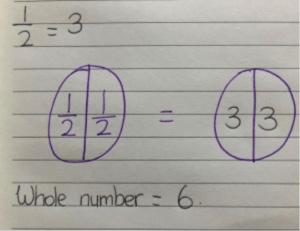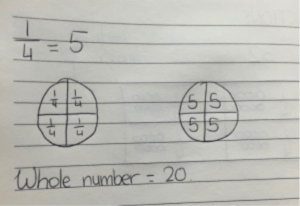# Teaching Fractions (3rd Class)

Some strategies for teaching fractions!

Paper Folding

I showed the children a piece of A4 paper and asked ‘I fold this page into two pieces. What is each piece called?’ Most children knew – half. So I asked how do you write ‘half’ and a child came to the board and wrote half. I then asked how to write it using numbers and another child wrote that -1/2.

I gave each child a piece of A4 paper which they folded in half and labelled 1/2. (each side). Then they coloured each side in a different colour.

Next they folded the same piece of paper into quarters (halved their half) and we discussed how many parts and labelled these sections as quarters (1/4).

Then we compared the two – how many quarters make a half? Which is bigger a half or a quarter? etc.Paper folding works well and enables the children to see the link between halves, quarters and eights.Shapes

I gave the children a number of different shapes which they folded into halves, quarters, eights etc.

Find a fraction of a number

The children already knew some halves and quarters. By using the paper folding – they understood that to find quarter – they could half the number and then half again. (To find eights – they could half, then half it and finally half it again.)

We used counters and divided them out on the paper to find half/quarter/ eights of a number.

This shows how the children divide the counters and can then count how many in 1 quarter/2 quarters/ 3 quarters etc.Find the whole numberI found drawing the circles showing quarter and then replacing it with the number helped the children to understand how they would find the whole number.

Some children realised that they could just multiply to find the whole number but others prefer to draw and fill in the numbers so they can find the whole number.

Equivalent Fractions

When teaching equivalent fractions – draw a circle or rectangle and divide it into different ways – halves, quarters, and eights. Colour the circle in the same way and the children will then see the equivalent fractions.This site uses Akismet to reduce spam. Learn how your comment data is processed.